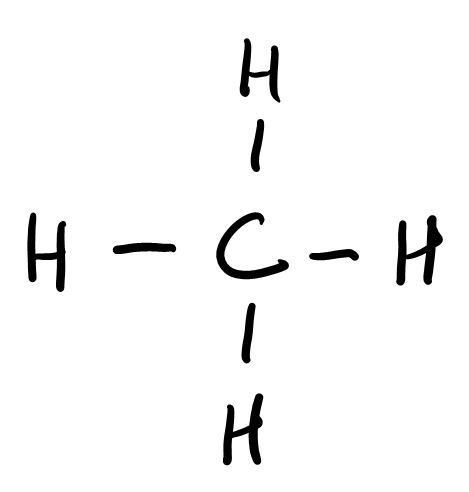# 1.6.1: Sample exam 1

$$\newcommand{\vecs}{\overset { \rightharpoonup} {\mathbf{#1}} }$$ $$\newcommand{\vecd}{\overset{-\!-\!\rightharpoonup}{\vphantom{a}\smash {#1}}}$$$$\newcommand{\id}{\mathrm{id}}$$ $$\newcommand{\Span}{\mathrm{span}}$$ $$\newcommand{\kernel}{\mathrm{null}\,}$$ $$\newcommand{\range}{\mathrm{range}\,}$$ $$\newcommand{\RealPart}{\mathrm{Re}}$$ $$\newcommand{\ImaginaryPart}{\mathrm{Im}}$$ $$\newcommand{\Argument}{\mathrm{Arg}}$$ $$\newcommand{\norm}{\| #1 \|}$$ $$\newcommand{\inner}{\langle #1, #2 \rangle}$$ $$\newcommand{\Span}{\mathrm{span}}$$ $$\newcommand{\id}{\mathrm{id}}$$ $$\newcommand{\Span}{\mathrm{span}}$$ $$\newcommand{\kernel}{\mathrm{null}\,}$$ $$\newcommand{\range}{\mathrm{range}\,}$$ $$\newcommand{\RealPart}{\mathrm{Re}}$$ $$\newcommand{\ImaginaryPart}{\mathrm{Im}}$$ $$\newcommand{\Argument}{\mathrm{Arg}}$$ $$\newcommand{\norm}{\| #1 \|}$$ $$\newcommand{\inner}{\langle #1, #2 \rangle}$$ $$\newcommand{\Span}{\mathrm{span}}$$

This is a 50 minute exam, i.e. you have about 10 minutes for each page.

### Page 1 (20 pts)

1.     Which of the two units for measuring mass is bigger, kilogram (kg) or gram (g)?

2.     If you convert a mass from kg to g, will the number get bigger or smaller?

3.     What are the two conversion factors (fractions) relating kg and g?

1 = —————                          1 = ————

4.     How much is a mass of 0.040 kg in grams? Use your answer from 2) to check your work

m = 0.040 kg  =

5.     In the “oxygen in air” lab experiment, you measured the volume of air in a graduated cylinder held upside down and submerged in water. If you did your reading 10 m below the water level (where the pressure is much higher than atmospheric pressure), would you record a volume that is lower or higher than expected? Use Boyle’s law to explain your answer.

### Page 2 (20 pts)

6.     Give an example of an element and a compound that you encounter in your daily life

a.      element:

b.     compound:

7.     What do elements and compounds have in common?

8.     What is the difference between an element and a compound?

9.     Complete the following statements about subatomic particles:

The atomic number is the number of ___________________

The mass number is the sum of ____________________________

10.     Complete the following nuclear reaction equation to figure out which product the alpha decay of Au-185 will yield.

$\ce{^185_79Au -> ^4_2He +}$

### Page 3 (20 pts)

Questions 11-14 refer to the following equation, which shows what happens to mercury(II) oxide when heated:

$\ce{2HgO(s) -> 2Hg(s) + O2(g)}$

11.     Is the reactant an element or a compound? Explain.

12.     What do the labels (g) and (s) represent?

13.     Do you think the volume will change? Explain.

14.     Do you think the mass will change? Explain.

15.     Write the chemical formula for the following compounds (for ionic compounds, first show into which ions it dissociates, as in the example; for covalent compounds, write covalent and then its name, as in the example):

Sodium chloride                     Na+ + Cl-                              NaCl

Hydrogen chloride                  covalent                      HCl

a.      Dinitrogen tetroxide

b.     Magnesium chloride

c.      Potassium phosphate

d.     Nitrogen trichloride

### Page 4 (20 pts)

16.     Ammonia, NH3, is a covalent compound. Draw the Lewis structure of ammonia.

17.     Formaldehyde, H2CO, is a covalent compound. Draw its Lewis structure.

18.     What shape does the water molecule have? Explain (the Lewis structure is shown below).19.     What shape does the methane molecule have? Explain (the Lewis structure is shown below).### Page 5 (20 pts)

20.     What is the molar mass of benzaldehyde, C7H6O, given that the molar mass of carbon, oxygen and hydrogen atoms is 12.0 g/mol, 16.0g/mol and 1.0 g/mol, respectively?

M =

21.     What is the mass of n = 0.20 mol of benzaldehyde? Please show how the units cancel out in the calculation, and make sure that the remaining units are appropriate for a mass.

m =

22.     DNA, the molecule carrying the genetic information we inherit from our parents, contains phosphorous. Radioactive 32P isotopes are used in determining the sequence of DNA, and its half-life of 32P is 14 days. If you have 20 ng of 32P waste, how long would you have to wait until less than 1 ng radioactive material is left? (Make a table showing the activity after 2 weeks, 4 weeks and so on).

1.6.1: Sample exam 1 is shared under a CC BY-NC-SA 4.0 license and was authored, remixed, and/or curated by LibreTexts.